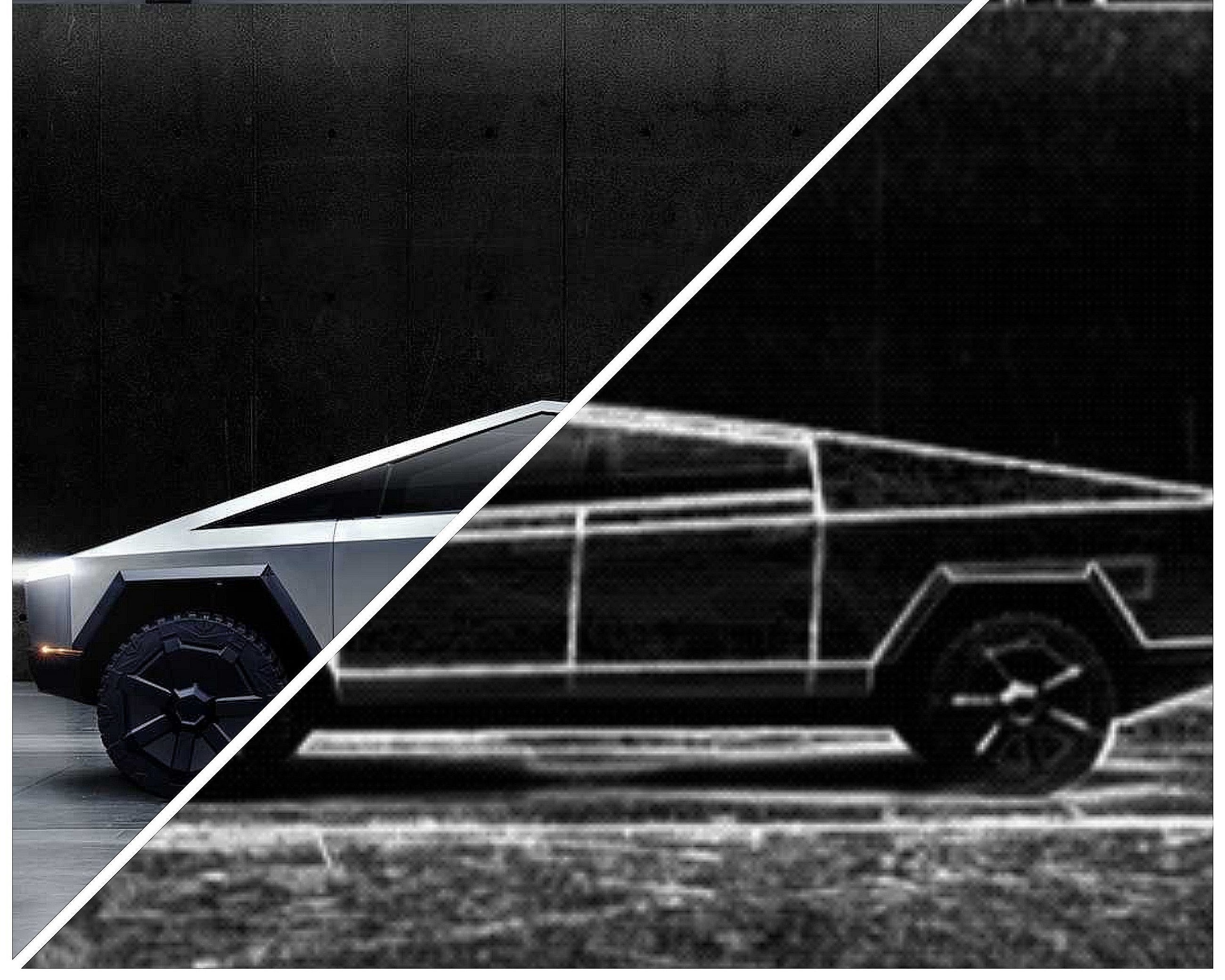# Convolutional Neural Networks

Original article was published by Brighton Nkomo on Artificial Intelligence on Medium# (Part 1: Edge Detection)

When I was doing an online convolutional neural network course from the deep learning specialization on Coursera by Andrew Ng, I noticed that there there are no slides, there are no given notes and there is no prescribed textbook . My series of blog post aims to summarize what was discussed in the CNN course 4/5 in the specialization as simple as possible.

So what is a convolutional neural network? A convolutional neural networks (CNN or ConvNet) is a type of deep learning neural network, usually applied to analyzing visual imagery whether it’s detecting edges in the image (vertical edges, horizontal edges, 45 degree angled edges etc). CNNs are applied in image and video recognition, recommender systems, image classification, medical image analysis, natural language processing, and financial time series.

# 1. Vertical Edge Detection

The purpose of detecting sharp changes in image brightness is to capture important events and changes in properties of the world.

Suppose that you want to detect vertical edges on the cyber-truck image. How would you detect vertical edges? To simplify this problem, it would be best to consider a simpler problem.

The grid on the left in figure 1 above represents a gray scale image with a 6 by 6 resolution. The numbers are the intensity values, the brighter the image the higher the value and vice versa. Because this is a grayscale image, this is just a 6 by 6 by 1 matrix rather than 6 by 6 by 3 when it’s a color image with 3 separate channels (the red, green and blue channels).

In order to detect edges or lets say vertical edges in his image, what you can do is construct a 3 by 3 matrix and in the terminology of convolutional neural networks, this is going to be called a filter (sometimes research papers will call this a kernel instead of a filter but I am going to use the filter terminology in this blog post).

And what you are going to do is take the 6 by 6 image and convolve it (the convolution operation is denoted by this asterisk) and convolve it with the 3 by 3 filter.

The output of convoluting the 6 by 6 matrix with a 3 by 3 matrix will be a 4 by 4 matrix. The way you compute this 4 by 4 output is as follows, to compute the first elements, the upper left element of this 4 by 4 matrix, what you are going to do is take the 3 by 3 filter and paste it on top of the 3 by 3 region of your original input image. Notice the convolution matrix entries (1, 1, 1, 0, 0, 0, -1, -1, -1) are written in the top right corners of the blue region and circled in green.

And what you should do is take the element wise product of the entries in the blue 3 by 3 region and corresponding the filter matrix entries which are circles in green. Then add them all up and you should get -5. This -5 value will be the first entry of the 4 by 4 output as shown in figure 2 on the right.

Next, to figure out what is this second entry is, you are going to take the blue square and shift it one step to the right like so and you are going to do the same element wise product and then addition. You do the same for the third , fourth entries and so on as illustrated by GIF 1 below.

So why is this doing vertical edge detection? Lets look at another example.